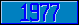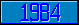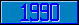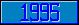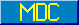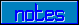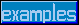## \$%ARCCOT

### M[UMPS] by Example

```
2-Jul–96, 6:54:35

Routine Save for Trigonometry Library function \$%ARCCOT^MATH

;

; Unless otherwise noted, the code below

; was approved in document X11/95–12

;

; If corrections have been applied,

; first the original line appears,

; with three semicolons at the beginning of the line.

;

; Then the source of the correction is acknowledged,

; then the corrected line appears, followed by a

; line containing three semicolons.

;

ARCCOT(X,PREC) ;

Set PREC=\$Get(PREC,11)

Set X=1/X

Quit \$%ARCTAN^MATH(X,PREC)

;===

;

;

;

```